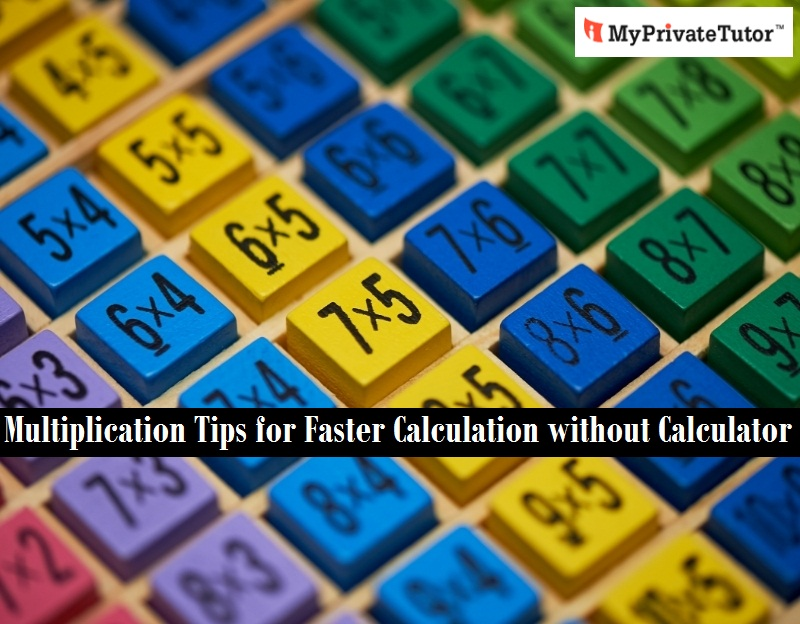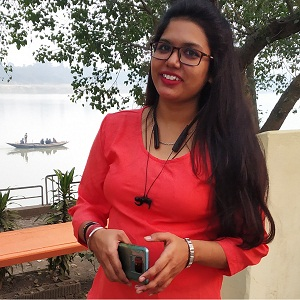# Multiplication Tips for Faster Calculation without Calculator

Apr 21, 2021There are a number of exams where the use of a calculator is allowed. But till a certain level of education, a calculator is restricted and the candidates have to calculate large numbers on their own.

Even if we skip the exams of global levels, different board exams, as well as final exams of different classes, include large numbers. To make the exam complicated, the examiners include larger digits. The students need to calculate these without any calculator. But, there are some tips to help these learners to calculate large numbers. Here, a few calculation tips are included.

### Multiplication Below 10

For the students of any class, it becomes great trouble to remember the tables. For them, it can be a master tip; however, it may need some time for them to learn this tip accurately and use it for fast calculation. But, once they have learned this, no multiplication will be a problem for them.

Let's take an example here, 8 x 9

To calculate this multiplication using this tip the first step is to find out what makes both the numbers 10. We will write it like this

• 8 x 9
• (2) (1)

The next step is to substract any one of these numbers in the round bracket with the number diagonally above it. It means you either have to do 9 - 2 or 8 - 1. The answer will anyway be the same. It will be 7. This will be the first digit of your original multiplication. Second digit will be the product of the digits in round bracket which is 2 x 1 = 2. The final product will be 8 x 9 = 72.

Let's have a look at another example:

• 7 x 7
• (3) (3)
• 7 - 3 = 4
• 3 x 3 = 9
• So, 7 x 7 = 49

### Multiplication of Teens

To a huge number of students, tables of teens seem impossible to by heart. For them, this is an excellent tip. Let's begin with an example:
15 x 17

Here we need to write how much these numbers are from 10. We will write it like this:

• 15 x 17
• (5) (7)

In the next step, add either of numbers in the round bracket with the numbers diagonally above them. So, either consider 15 + 7 or 17 + 5. In both cases, answer will be 22.

Now put a 0 after this number which makes it 220 and add the product of the digits in the round bracket above 5 x 7 = 35. 220 + 35 = 255.

Another example will make it clear:

• 12 x 19
• (2) (9)
• 12 + 9 or 19 + 2 = 21
• Adding a 0,makes it 210
• Adding the product of 2 and 9 or 18, makes it 210 +18 = 228. Or 12 x 19 = 228.

### Multiplication of Any Number between 10 and 100

Here, we are taking 2 numbers 81 and 96. First, we need to check how less these numbers are from 100.

• 81 x 96
• (19) (4)

Subtract the numbers in the round bracket from the numbers diagonally above them. Either take 81 - 4 or 96 - 19. Answer will be 77 in both. This is the first part of your answer.
Next, obtain the product of the digits in brackets - 19 x 4 = 76. This is the second part of the answer. So, the answer is 81 x 96 = 7776.

Let's take another example:

• 95 x 88
• (5) (12)
• 95 - 12 or 88 - 5 = 83
• 5 x 12 = 60

### Multiplication of Numbers Above 100

To multiply numbers above 100, you need to first understand how much the number is than 100.

Let's take an example on how to write this:

• 115 x 108
• (15) (8)

Add these numbers with the numbers diagonally above them. Either 115 + 8 or 108 + 15 = 123. Multiply it with 100.

Now it is 123 x 100 = 12300 and add product of the numbers in brackets. 15 x 8 = 120. Final answer after addition is 12300 + 120 = 12420.

Another example will make the process clearer:

• 125 x 107
• (25) (7)
• 125 + 7 or 107 + 25 = 132
• Multiplication by 100 makes it 13200.
• Adding the product of 25 and 7 or 175 makes it 13200 + 175 = 13375.

So, it will not be a great deal to multiply these numbers for the candidates who cannot remember the tables. They simply need to follow these rules while multiplying. Alternatively, they can hire a math tutor to learn some more tricks to multiply different numbers.

Article Posted in: Academics How-Tos and Tips### Rachita

A blogger turned content editor, Rachita curates the blogs for MyPrivateTutor Thailand. She loves researching and she is fascinated by the unusual facts that come by. Apart from education and e-Learning, she loves to write on sustainable fashion, food, movies, and social media trends.